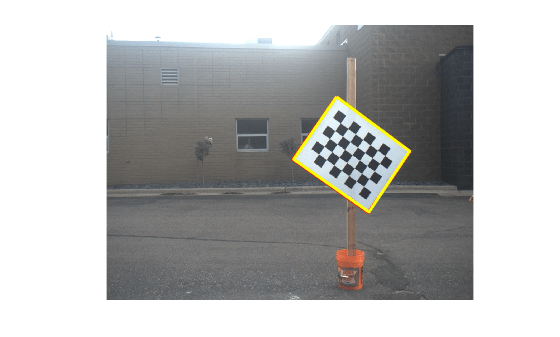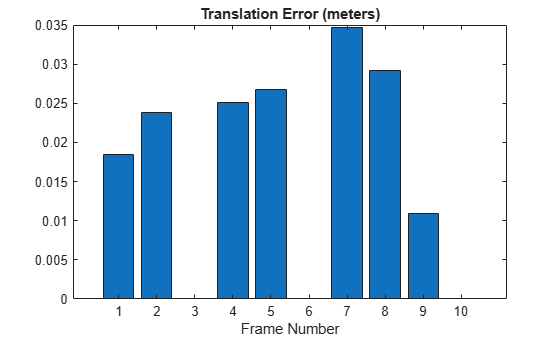# estimateLidarCameraTransform

Estimate rigid transformation from lidar sensor to camera

## Syntax

``tform = estimateLidarCameraTransform(ptCloudPlanes,imageCorners3d)``
``[tform,errors] = estimateLidarCameraTransform(___)``
``[___] = estimateLidarCameraTransform(___,Name,Value)``

## Description

````tform = estimateLidarCameraTransform(ptCloudPlanes,imageCorners3d)` estimates the transformation between a lidar sensor and a camera using the checkerboard calibration pattern features extracted from each sensor.```
````[tform,errors] = estimateLidarCameraTransform(___)` returns the inaccuracy in estimating the transformation matrix `errors` using the input arguments from the previous syntax.```

example

````[___] = estimateLidarCameraTransform(___,Name,Value)` specifies options using one or more name-value pair arguments in addition to any combination of arguments in previous syntaxes. For example, `'Verbose',true` sets the function to display progress.```

## Examples

collapse all

Estimate the rigid transformation from a lidar sensor to a camera using data captured from the lidar sensor and camera calibration parameters. Use these three steps:

1. Load the data into the workspace.

2. Extract the required features from images and point cloud data.

3. Estimate the rigid transformation using the extracted features.

Load images and point cloud data into the workspace.

```imageDataPath = fullfile(toolboxdir('lidar'),'lidardata',... 'lcc','vlp16','images'); imds = imageDatastore(imageDataPath); imageFileNames = imds.Files; ptCloudFilePath = fullfile(toolboxdir('lidar'),'lidardata',... 'lcc','vlp16','pointCloud'); pcds = fileDatastore(ptCloudFilePath,'ReadFcn',@pcread); pcFileNames = pcds.Files;```

Load camera calibration files into the workspace.

```cameraIntrinsicFile = fullfile(imageDataPath,'calibration.mat'); intrinsic = load(cameraIntrinsicFile);```

Feature Extraction

Specify the size of the checkerboard squares in millimeters.

`squareSize = 81;`

Estimate the checkerboard corner coordinates for the images.

```[imageCorners3d,planeDimension,imagesUsed] = estimateCheckerboardCorners3d( ... imageFileNames,intrinsic.cameraParams,squareSize);```

Filter the point clouds based on the images used.

`pcFileNames = pcFileNames(imagesUsed);`

Detect the checkerboard planes in the filtered point clouds using the plane parameters `planeDimension`.

```[lidarCheckerboardPlanes,framesUsed] = detectRectangularPlanePoints( ... pcFileNames,planeDimension,'RemoveGround',true);```

Extract the images, checkerboard corners, and point clouds in which you detected features.

```imageFileNames = imageFileNames(framesUsed); pcFileNames = pcFileNames(framesUsed); imageCorners3d = imageCorners3d(:,:,framesUsed);```

Estimate Transformation

Estimate the transformation using checkerboard planes from the point clouds and 3-D checkerboard corner points from the images.

```[tform,errors] = estimateLidarCameraTransform(lidarCheckerboardPlanes, ... imageCorners3d,'CameraIntrinsic',intrinsic.cameraParams);```

Display translation, rotation, and reprojection errors as bar graphs.

```figure bar(errors.TranslationError) xlabel('Frame Number') title('Translation Error (meters)')``````figure bar(errors.RotationError) xlabel('Frame Number') title('Rotation Error (degrees)')``````figure bar(errors.ReprojectionError) xlabel('Frame Number') title('Reprojection Error (pixels)')```## Input Arguments

collapse all

Segmented checkerboard planes, specified as a `pointCloud` object or P-by-1 array of `pointCloud` objects. P is the number of point clouds. Each `pointCloud` object must contain points that represent a checkerboard (rectangular) plane.

P must be equal for both the `ptCloudPlanes` and `imageCorners3d` arguments. This means that number of point clouds and number of images used for detection must also be equal.

3-D coordinates of the checkerboard corners, specified as a 4-by-3 matrix or 4-by-3-by-P array. P represents the number of camera images used for detection. Each row of a channel contains the 3-D coordinates, in the form of [x,y,z], of a checkerboard corner in meters extracted from the corresponding camera image. P must be equal for both the `ptCloudPlanes` and `imageCorners3d` arguments. This means that number of point clouds and number of images used for detection must also be equal.

Data Types: `single` | `double`

### Name-Value Pair Arguments

Specify optional comma-separated pairs of `Name,Value` arguments. `Name` is the argument name and `Value` is the corresponding value. `Name` must appear inside quotes. You can specify several name and value pair arguments in any order as `Name1,Value1,...,NameN,ValueN`.

Example: `'Verbose',true` sets the function to display progress.

Checkerboard corners in the lidar frame, specified as the comma-separated pair consisting of `'Lidar3DCorners'` and a 4-by-3-by-P array where P is the number of point clouds.

If the user specifies the checkerboard corners in the lidar frame, then the function does not calculate them internally.

Data Types: `single` | `double`

Initial rigid transformation, specified as the comma-separated pair consisting of `'InitialTransformation'` and a `rigid3d` object.

The function assumes the rotation angle between the lidar sensor and the camera is in the range [-45 45] along each axis. For any other range of the rotation angle, use this name-value pair to specify an initial transformation to improve function accuracy.

Camera intrinsic parameters, specified as the comma-separated pair consisting of `'CameraIntrinsic'` and a `cameraIntrinsics` object or `cameraParameters` object.

Display function progress, specified as the comma-separated pair consisting of `'Verbose'` and a logical `0` (`false`) or logical `1` (`true`).

Data Types: `logical`

## Output Arguments

collapse all

Lidar to camera rigid transformation, returned as a `rigid3d` object. The returned object registers the point cloud data from a lidar sensor to the coordinate frame of a camera.

Inaccuracy of the transformation matrix estimation, returned as a structure. The structure contains these fields.

• `RotationError` — The difference between the normal angles defined by the checkerboard planes in the point clouds (lidar frame) and those in the images (camera frame). The function estimates the plane in the image using the checkerboard corner coordinates. The function returns the error values in degrees, as a P-element numeric array. P is the number of point clouds.

• `TranslationError` — The difference between the centroid coordinates of checkerboard planes in the point clouds and those in the images. The function returns the error values in meters, as a P-element numeric array. P is the number of point clouds.

If you specify camera intrinsic parameters to the function using `'CameraIntrinsic'` name-value pair, then the structure contains this additional field.

• `ReprojectionError` — The difference between the projected (transformed) centroid coordinates of the checkerboard planes from the point clouds and those in the images. The function returns the error values in pixels, as a P-element numeric array. P is the number of point clouds.

Data Types: `struct`

Introduced in R2020b

## SupportGet trial now